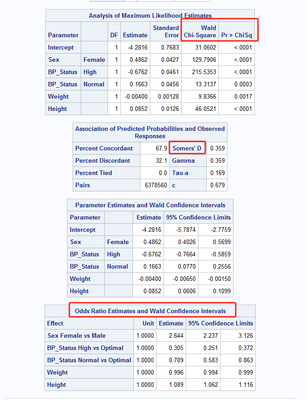## Logistic regression Analysis

Hi Team,

I have a new request to create a statistics table with logistic regression. I have attached a sample with this for reference

Expect Somme insights on this since Iam new to this scenario

Covariate Estimate odds ratio 95%ci waldchisqr pvalue

These needs to find
9 REPLIES 9Ksharp
Super User

## Re: Logistic regression Analysis

``````proc logistic data=sashelp.heart;
class sex bp_status;
model status=sex bp_status weight height/clparm=wald clodds=wald ;
/*oddsratio sex/cl=wald;*/
run;``````## Re: Logistic regression Analysis

Hi I have found a sample code, but i needed the below results for my requiremnt. I am not understanding how to pass the proper variables and options . the requirement below

 Covariate Estimate Odds Ratio 95% CI Wald Chi-Square P-value Results xx.xx xx.xx xx.xx xx.xx xx.xx Male xx.xx xx.xx xx.xx xx.xx xx.xx Female Reference Reference Reference Reference Reference Results*Male xx.xx xx.xx xx.xx xx.xx xx.xx Results*Female Reference Reference Reference Reference Reference

## Re: Logistic regression Analysis

This is basic output from PROC LOGISTIC. But what you want appears in two separate tables. Below assumes that your binary response is called Response, and has values 0 or 1 with 1 being the event level of interest.

``````proc logistic data=YourDataSet;
class gender(ref='Male');
model Response(event="1")=Results gender Results*gender / clparm=wald;
run;
``````

## Re: Logistic regression Analysis

Hi I have used the code,.. the problem is that I am not able to get the ODDS ratio

## Re: Logistic regression Analysis

Hi I have used the code,.. the problem is that I am not able to get the ODDS ratio

--
Paige Miller

## Re: Logistic regression Analysis

Please find the data and the code below which i have.

``````ods trace on;
ods output Association=or2 GlobalTests=GBLT_2;

proc logistic data=mydata  outmodel=stat_3;
class gender (ref="F");
model improvement(event="Y")=result gender gender*result  / clodds=wald;
run;
ods trace off;``````

 improvement Result Gender Y 12 M N 13 F Y 14 M Y 15 F Y 16 M Y 17 M Y 18 F

## Re: Logistic regression Analysis

### Maxim 2

Everything you need to know about your program is in the log. Interpreting messages and NOTEs is essential in finding errors.

The log says:

``````NOTE: The CLODDS= option does not compute odds ratios for effects involved in interactions or nestings.
Specify the ODDSRATIO statement to compute odds ratios for these effects.

``````

So you need to add the ODDSRATIO command into your code. Maybe like this:

``````proc logistic data=mydata  outmodel=stat_3;
class gender (ref="F");
model improvement(event="Y")=result gender gender*result  / clodds=wald;
oddsratio gender;
oddsratio result;
run;``````

The log also says

```WARNING: There is a complete separation of data points. The maximum likelihood estimate does not exist.
WARNING: The LOGISTIC procedure continues in spite of the above warning. Results shown are based on the last maximum likelihood iteration. Validity
of the model fit is questionable.
```

which means that the results you get are questionable.

--
Paige MillerKsharp
Super User

## Re: Logistic regression Analysis

As Paige said using ODDSRATIO statement.

proc logistic data=sashelp.heart(obs=1000);
class sex bp_status;
model status=sex bp_status weight height sex*height/clparm=wald clodds=wald ;
oddsratio height/at(sex=all) cl=wald;
run;
Discussion stats
• 9 replies
• 398 views
• 0 likes
• 5 in conversation